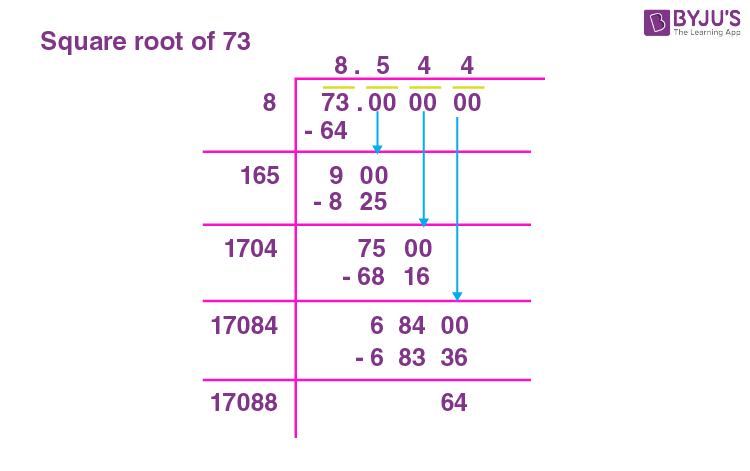# Square Root of 73

The square root of 73 is irrational. The approximate value of the square root of 73 is 8.54. When 8.54 is multiplied by 8.54, the product is 72.9, which when rounded off will be 73. Since 8.54 is multiplied by itself, 8.54 is the square root of 73. In general, when an integer “i” is multiplied with the same integer “i”, the resultant is called the perfect square and the integer “i” is its square root. i.e., If ± i × ± i = p, then i is the square root of p.

The given number 73 lies between 64 and 81, where 64 is obtained by the square of 8 ( ±8 × ±8 = 64) and 81 is obtained by multiplying 9 with 9. (±9 × ±9 = 81).

This means, that 73 is not a perfect square and does not have a perfect square root. Hence, the square root of 73, √73 is an irrational number. You can refer to Finding Square root with Example for details.

Note the Following:

• The Square root of 73 = √73 = 2√73, where ‘√’ is radical, 73 is the radicand and 2 is the index
• Exponential Form of Square root of 73 = 731/2
• Solution for √73 ≈ 6.2
• The square root of 73 is Irrational = True

## What is the Square root of 73?

The square root of 73 is 6.2 as the square root of 73 is irrational.

 √73 =8.544

## How to Find the Square root of 73?

There are three methods to find the Square root of 73

• Prime Factorisation method
• Long Division method
• Repeated Subtraction method

### Square root of 73 by Prime Factorisation Method

In the prime factorization method, the following steps are followed.

1. Divide 73 with prime factors. Since 73 is a prime number, there are no other factors than 1 and 73.

Hence the square root of 73 is √73, which is irrational.

The given number, 73 will be expressed as;

 73 73 × 1

### Square root of 73 by Long Division Method

In the Long Division method, for 73, divide 73 by selecting a divisor such that d × d is less than or equal to 73. If d = 73, then d is the perfect square root of 73, it is irrational.

Choosing d from the list would be, the squares are

8 × 8 = 64

9 × 9 = 81Hence, 73 is not a perfect square. Therefore, 73 is an irrational number.

### Square root of 73 by Repeated Subtraction Method.

In the repeated Subtraction method, 73 is subtracted by odd numbers starting with 1, then 72 by 3, then 69 by 5 and so on. If these subtraction steps result in zero, then 73 will be a perfect square number, and the step at which zero is the remainder, its square root.

The table below shows repeated subtraction for the given number 73.

 Step 1 73 – 1 = 72 Step 2 72 – 3 = 69 Step 3 69 – 5 = 64 Step 4 64 – 7 = 57 Step 5 57 – 9 = 48 Step 6 48 – 11 = 37 Step 7 37 – 13 = 24 Step 8 24 – 15 = 9 Step 9 9 – 17 = -8

Since the difference does not become zero, 73 is not a perfect square number and hence it does not have a perfect square root. The root lies between 7 and 8, resulting in a decimal root.

## Video Lessons

### Visualising square roots### Finding Square roots## Solved Examples

1. Which number is that lesser than or greater than 73, that has a perfect square root?

64, which is less than 73 and 81, which is greater than 73, are the perfect square numbers. 8 and 9 are their perfect square roots, respectively.

2. What is the square root of 100?

10 is the square root of 100.

## Frequently Asked Questions on Square root of 73

### What is the Square root of 73?

The Square root of 73 is 8.54

### 73 is a perfect square number. True or False?

False. 73 is not a perfect square number.

### What are the factors of 73?

The factors of 73 are 1 and 73.

### Simplify √73?

√73 = √(1 × 73). No further simplification is possible.

### The square root of 73 is a rational number. True or False?

False. The square root of 73 is not a rational number.